# Finding Periods in Signal Equation Through Visualization

in #engineering2 years ago

Back in high school and university in department of science and mathematic, it is compulsory to solve mathematical problem manually using theories through formulas. However in Engineering, it is the application that is important, and thus we are encouraged not to waste time solving manually and instead use simulators such as Matlab, Octave, and today there are online ploters. For example in one of my assignments in Signal and System Analysis course, I was asked to find periods in signal equations through visualization rather solving through formulas. Period is defined as a complete cycle.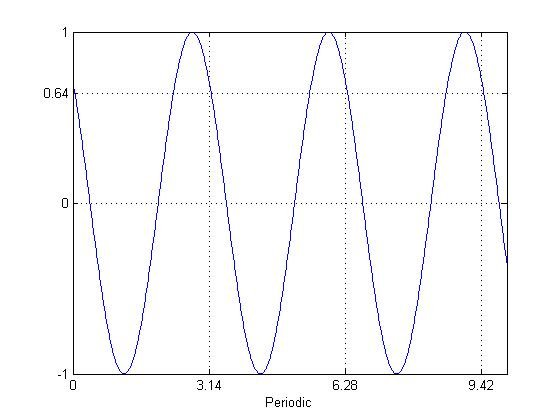x(t)=cos⁡(2t+π/4), Period = π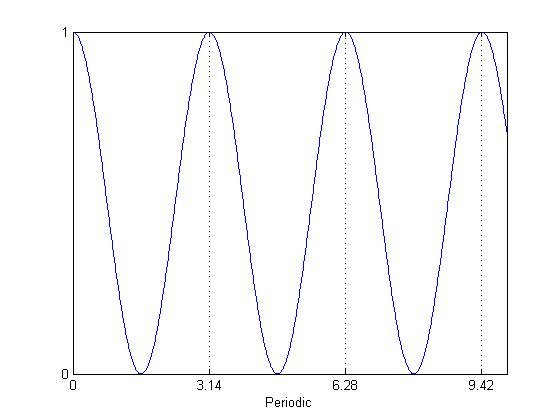x(t)=(cos⁡(t))2, Period = π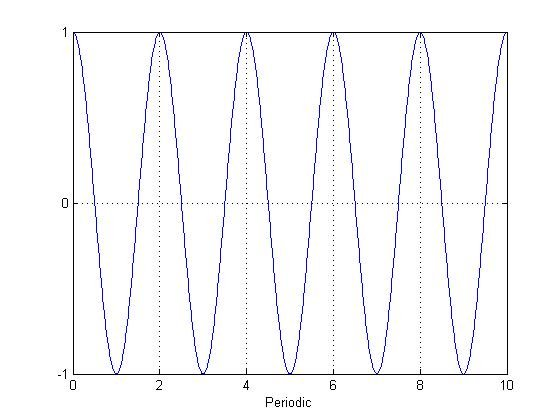x(t)= ejπt, Period = 2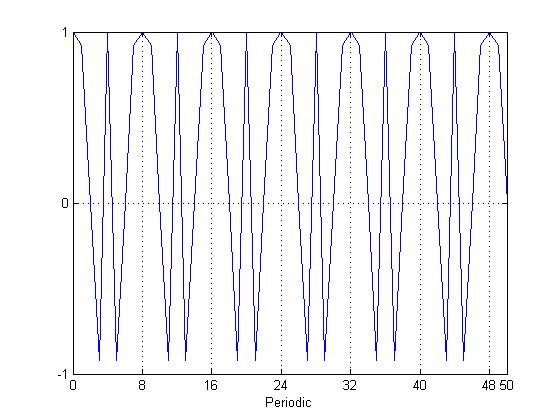x[n]=cos⁡((πn2)/8), Period = 8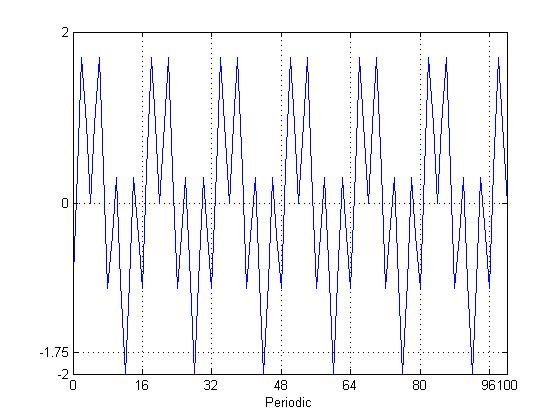x[n]=cos⁡[πn/4]+sin⁡[πn/8]-2cos⁡[πn/2], Period = 16

## Mirrors

Sort:

Congratulations @fajar.purnama! You have completed the following achievement on the Hive blockchain and have been rewarded with new badge(s) :You received more than 1000 as payout for your posts. Your next target is to reach a total payout of 2000

You can view your badges on your board and compare yourself to others in the Ranking
If you no longer want to receive notifications, reply to this comment with the word `STOP`